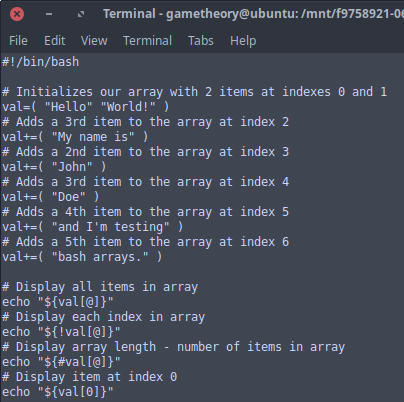# Bash ArraysBash arrays are quite powerful and robust, but not often used in the command line. They are variables which contain multiple values often referred to as items or elements. Arrays do not have a maximum limit for items they can contain nor do the values need to be assigned or indexed contiguously.

## Writing and Using Bash Arrays

The first item in an array is indexed at position zero “0”. You can add multiple items to an array in one variable or through multiple variables.

In this example we have an array variable with 2 items, each quoted:

``val=( "Hello" "World!" )``

Here we add 5 more items to the array:

``````val+=( "My name is" )
val+=( "John" )
val+=( "Doe" )
val+=( "and I'm testing" )
val+=( "bash arrays." )``````

Notice the plus “+” sign.

If you’re following, between the 2 examples our array now has 7 items in it.

Display all items in the array:

``echo "\${val[@]}"``

Output:

``Hello World! My name is John Doe and I'm testing bash arrays.``

Display each index in the array:

Here we add and exclamation “!” symbol to retrieve the indexes.

``echo "\${!val[@]}"``

Output:

Looking at the output, you can see there’s 7 items.

``0 1 2 3 4 5 6``

Display the array length:

This will give you the sum of items in the array.

``echo "\${#val[@]}"``

Output:

``7``

Display items by index position:

Here we reference items 3 and 4.

``````echo "\${val}"
echo "\${val}"``````

Output:

``John Doe``

Capitalize the first letter in an item:

For this next example we’re going to use the last item in the array at index 6. Notice the single caret “^” symbol. This capitalizes the first letter in the array.

``echo "\${val^}"``

Output:

``Bash arrays``

Capitalize every letter in the array:

Notice the double caret “^^” symbol. This will capitalize every letter in the array.

``echo "\${val[@]^^}"``

Output:

``HELLO WORLD! MY NAME IS JOHN DOE AND I'M TESTING BASH ARRAYS.``

Make the first letter in an item lowercase:

We will now use item 3 for this example. Notice the comma “,”.

``echo "\${val,}"``

Output:

``john``

Make every letter in the array lowercase:

Notice we are now using double commas “,,”.

``echo "\${val[@],,}"``

Output:

``hello world! my name is john doe and i'm testing bash arrays.``

Using a for loop to display all items in the array:

In this for loop we will iterate through every item using their index as we previously covered in this tutorial.

Take notice how we use the “\$i” variable.

``````for i in \${!val[@]}; do
echo "\${val[\$i]}"
done``````

Output:

Each item is displayed on its own line.

``````Hello
World!
My name is
John
Doe
and I'm testing
bash arrays.``````

Here’s a complete script with a for loop to display our bash array.

``````#!/bin/bash

val=( "Hello" "World!" )
val+=( "My name is" )
val+=( "John" )
val+=( "Doe" )
val+=( "and I'm testing" )
val+=( "bash arrays." )

for i in \${!val[@]}; do
echo "\${val[\$i]}"
done``````

## Conclusion

This concludes the tutorial. There’s many useful ways to use arrays in bash. This was an introductory run through and only scratches the surface. Hope this gave you some ideas and new tricks for using bash arrays.

Tags: tutorials, linux